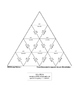# Triangle Puzzle - Two-Step Equations, Positive Coefficients, Integer SolutionsSubject
Resource Type
File Type
PDF (29 MB|4 pages)
Standards
\$1.00
• Product Description
• Standards
Students solve this puzzle by matching equations with their solutions.

In this puzzle, all equations have positive coefficients and integer solutions.

Included are two versions of the puzzle:
(1) the solution, which may also be printed and cut apart before giving to students; and
(2) a "mixed up" version of the puzzle, which you may distribute to students if you want them to cut the pieces apart themselves before solving!

The puzzle is sized to print on an 8.5x11 piece of paper, and each piece measures approximately 1.7 inches wide.
Solve linear equations and inequalities in one variable, including equations with coefficients represented by letters.
Total Pages
4 pages